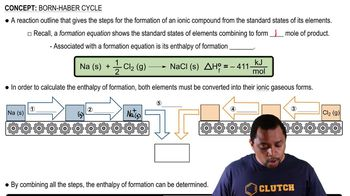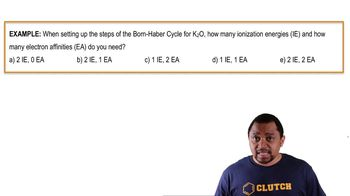Start typing, then use the up and down arrows to select an option from the list.
4:18 minutes
Problem 96
Textbook Question

# Use the following information plus the data given in Tables 6.2 and 6.3 to calculate the second electron affinity, Eea2, of oxygen. Is the O2-ion stable in the gas phase? Why is it stable in solid MgO? Heat of sublimation for Mg1s2 = +147.7 kJ/mol Bond dissociation energy for O21g2 = +498.4 kJ/mol Eea1 for O1g2 = -141.0 kJ/mol Net energy change for formation of MgO(s) from its elements = -601.7 kJ/molVerified Solution
This video solution was recommended by our tutors as helpful for the problem above.
65views